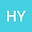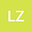The existence and nonexistence of global $L^2$-constrained minimizers for Kirchhoff equations with $L^2$-subcritical general nonlinearity
••Lina Zhang
Wuhan University of Science and Technology
Author Profile## Abstract

In this paper, we study the existence of global $L^2$-constrained minimizers related to the following Kirchhoff type equation: $$-\left(a+b\ds\int_{\R^N}|\nabla u|^2\right)\Delta u-f(u)=\lambda u,~~~x\in \R^N,~\lambda\in\R,$$ where $N\leq3$, $a,$ $b>0$ are constants, $f(u)$ is a general $L^2$-subcritical nonlinearity. By using the concentration compactness principle, we prove the sharp existence and nonexistence of global $L^2$-constraint minimizers.
12 Apr 2022Submitted to Mathematical Methods in the Applied Sciences
12 Apr 2022Submission Checks Completed
12 Apr 2022Assigned to Editor
12 May 2022Reviewer(s) Assigned
25 Aug 2022Review(s) Completed, Editorial Evaluation Pending
03 Sep 2022Editorial Decision: Revise Minor